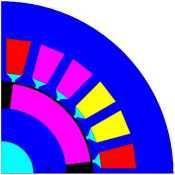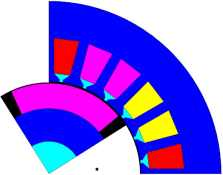# Quantity computation on points: about

## Definition

A computation session called Quantity computation on points enables the computation of spatial quantities in different computation points and at the same time:

• displays spatial quantities as arrows or squares in the graphic zone
• displays the values of point coordinates and the values of spatial quantities in a table

## General steps

A computation session on points proceeds as follows:

Stage Description
1

Opening of a computation session Quantity computation on points

2 Choice of the spatial quantity (or of the formula) to compute
Flux opens a dedicated context
3 Selection of a computation point

Display of the spatial quantity in the graphic zone

Display of values (coordinates of the point and values of the spatial quantity) in a table.

4 Repetition of the stage 3 for the different points
5 Closing of the session
The session is stored in the data tree.

## Computation point

A computation point is a spatial point, on which the user can evaluate a spatial quantity.

A computation point is defined by its coordinates and by its location in relation with the geometry, mesh, physics and kinematics (if there is a kinematic coupling).

## Spatial quantity

The spatial quantity depends on the activated physical application*.

The user can also use a formula with spatial quantities.

Note: * The detailed list of spatial quantities available for each physical application is presented in § Result analysis of each physical application.

## Graphical representation

According to the type of spatial quantity, the graphical representation in graphic zone is different, as presented below.

Quantity Graphical representation
Real scalar A colored square
Complex scalar A colored square
Real vector An arrow
Complex vector

An arrow

(with a cursor to change the phase represented in the graphic zone for steady state AC applications).

## Management of sessions

• It is possible to carry out as many sessions as the user wishes.
• All sessions of computation on points are automatically updated whenever the parameters configuration of the scenario is modified.
• Thus all the computed values of points computations correspond at the activated current parameters configuration of solved scenario.
• A session of Quantity computation on points is saved in the database of the Flux project. It is possible to open it again, to delete it or to fill it out by adding other computation points.
• It is possible to add one or several formula in an existing session.

If the user wishes to keep the results corresponding to a parameters configuration of the scenario, it will export the results before changing the parameters configuration.

## Similitude with a sensor

A sensor of the point type permits to compute almost the same quantities than with the quantity computation session on points , but with the following distinctions:

• no dedicated context, a sensor of the point type is defined via a simple dialog box
• no possibility to compute on several points at the same time, a sensor of the point type only permits to compute on a single point.

## Operation with mechanical set

When a new step is selected, the actualization of the session of computation on points does not modify the coordinates of the points of calculation.

If these points are situated within a mechanical set, they are not linked to the associated motion (see example below).

Stage Description
1

After the creation of the session of point computation, the created point is situated in a mobile mechanical set.

The calculation is validated.2

After the selection of a new step, the point does not change its coordinates.

The mechanical set has moved and the point is out of the mobile part.

As the point is attached to the mechanical set, the computation cannot be performed, and it is therefore invalid.